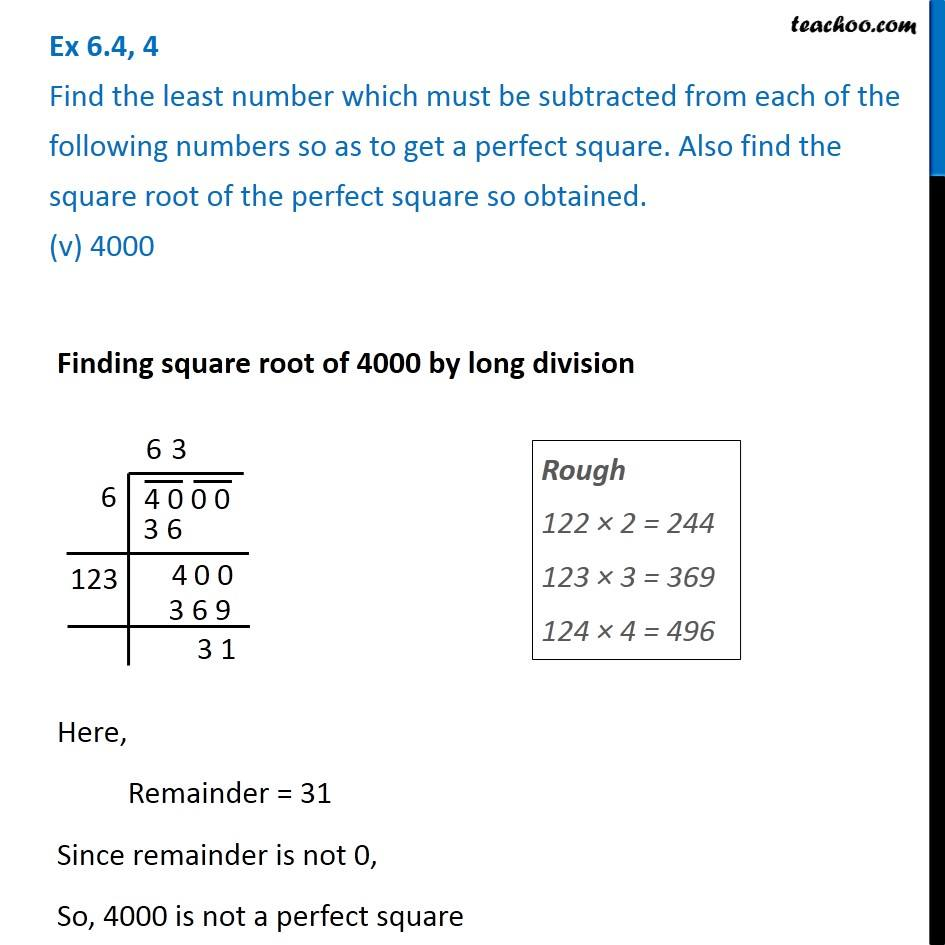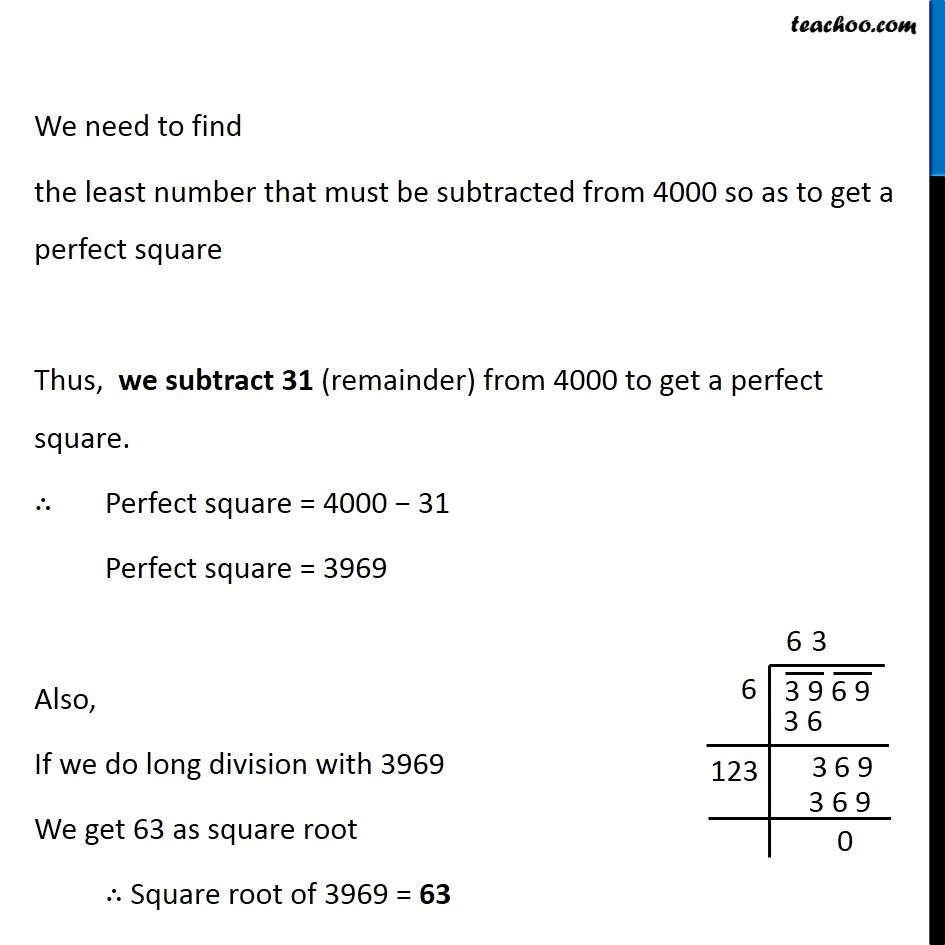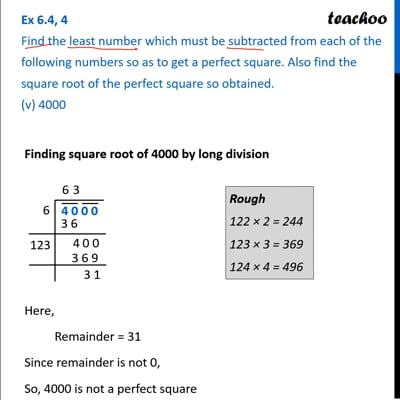Ex 6.4

Chapter 6 Class 8 Squares and Square Roots
Serial order wiseThis video is only available for Teachoo black users

### Transcript

Ex 6.4, 4 Find the least number which must be subtracted from each of the following numbers so as to get a perfect square. Also find the square root of the perfect square so obtained. (v) 4000 Finding square root of 4000 by long division Here, Remainder = 31 Since remainder is not 0, So, 4000 is not a perfect square Rough 122 × 2 = 244 123 × 3 = 369 124 × 4 = 496 We need to find the least number that must be subtracted from 4000 so as to get a perfect square Thus, we subtract 31 (remainder) from 4000 to get a perfect square. ∴ Perfect square = 4000 − 31 Perfect square = 3969 Also, If we do long division with 3969 We get 63 as square root ∴ Square root of 3969 = 63